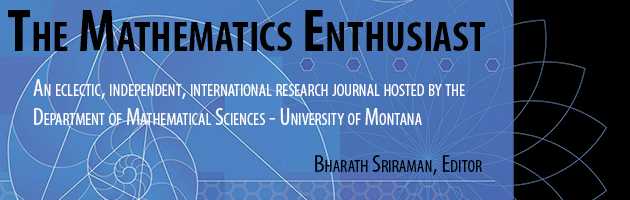•
•#### Article Title

Comparison of Geometric Figures

5

2-3

#### Abstract

Although the geometric equality of figures has already been studied thoroughly, little work has been done about the comparison of unequal figures. We are used to compare only similar figures but would it be meaningful to compare non similar ones? In this paper we attempt to build a context where it is possible to compare even non similar figures. Adopting Klein’s view for the Euclidean Geometry, we defined a relation “≤ ” as: S1 ≤ S2 whenever there is a Euclidean isometry f :R2 →R2 , so that . This relation is not an order because there are figures (subsets of R2 ) 1 2 S , S so that 1 2 S ≤ S , 2 1 S ≤ S and 1 2 S , S not geometrically equal. Our goal is to avoid this paradox and to track down non-trivial classes of figures where the relation “ ≤ ” becomes, at least, a partial order. For example there is no paradox if we restrict our attention just to compact figures; thus, we can compare a closed disc with a closed triangular region. Further we present some other “good” classes of figures and we extend our study to the Hyperbolic and to the Elliptic geometry. Eventually, there are still some open and quite challenging issues, which we present at the last part of the paper.

199

214

COinS